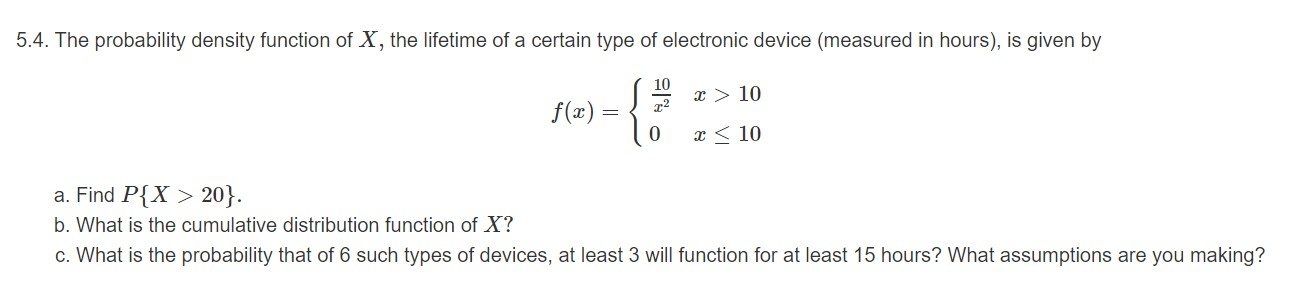# 5.4. The probability density function of X, the lifetime of a certain type of electronic device (measured in hours), is given by 10 x > 10 f) 0 10 a. Find P{X> 20}. b. What is the cumulative distribution function of X? c. What is the probability that of 6 such types of devices, at least 3 will function for at least 15 hours? What assumptions are you making?

Questionhelp_outlineImage Transcriptionclose5.4. The probability density function of X, the lifetime of a certain type of electronic device (measured in hours), is given by 10 x > 10 f) 0 10 a. Find P{X> 20}. b. What is the cumulative distribution function of X? c. What is the probability that of 6 such types of devices, at least 3 will function for at least 15 hours? What assumptions are you making? fullscreen

1 Rating

### Want to see this answer and more?

Experts are waiting 24/7 to provide step-by-step solutions in as fast as 30 minutes!*